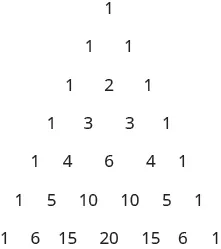Intermediate Algebra 2e

# Key Concepts

### Key Concepts

#### 12.1Sequences

• Factorial Notation
If n is a positive integer, then $n!n!$ is
$n!=n(n−1)(n−2)…(3)(2)(1).n!=n(n−1)(n−2)…(3)(2)(1).$

We define $0!0!$ as 1, so $0!=10!=1$
• Summation Notation
The sum of the first n terms of a sequence whose nth term $anan$ is written in summation notation as:
$∑i=1nai=a1+a2+a3+a4+a5+…+an∑i=1nai=a1+a2+a3+a4+a5+…+an$

The i is the index of summation and the 1 tells us where to start and the n tells us where to end.

#### 12.2Arithmetic Sequences

• General Term (nth term) of an Arithmetic Sequence
The general term of an arithmetic sequence with first term $a1a1$ and the common difference d is
$an=a1+(n−1)dan=a1+(n−1)d$
• Sum of the First n Terms of an Arithmetic Sequence
The sum, $Sn,Sn,$ of the first n terms of an arithmetic sequence, where $a1a1$ is the first term and $anan$ is the nth term is
$Sn=n2(a1+an)Sn=n2(a1+an)$

#### 12.3Geometric Sequences and Series

• General Term (nth term) of a Geometric Sequence: The general term of a geometric sequence with first term $a1a1$ and the common ratio r is
$an=a1rn−1an=a1rn−1$
• Sum of the First n Terms of a Geometric Series: The sum, $Sn,Sn,$ of the n terms of a geometric sequence is
$Sn=a1(1−rn)1−rSn=a1(1−rn)1−r$

where $a1a1$ is the first term and r is the common ratio.
• Infinite Geometric Series: An infinite geometric series is an infinite sum whose first term is $a1a1$ and common ratio is r and is written
$a1+a1r+a1r2+…+a1rn−1+…a1+a1r+a1r2+…+a1rn−1+…$
• Sum of an Infinite Geometric Series: For an infinite geometric series whose first term is $a1a1$ and common ratio r,
$If|r|<1,the sum is S=a11−r We say the series converges. If|r|≥1,the infinite geometric series does not have a sum. We say the series diverges.If|r|<1,the sum is S=a11−r We say the series converges. If|r|≥1,the infinite geometric series does not have a sum. We say the series diverges.$
• Value of an Annuity with Interest Compounded $nn$ Times a Year: For a principal, P, invested at the end of a compounding period, with an interest rate, r, which is compounded n times a year, the new balance, A, after t years, is
$At=P((1+rn)nt−1)rnAt=P((1+rn)nt−1)rn$

#### 12.4Binomial Theorem

• Patterns in the expansion of $(a+b)n(a+b)n$
• The number of terms is $n+1.n+1.$
• The first term is $anan$ and the last term is $bn.bn.$
• The exponents on a decrease by one on each term going left to right.
• The exponents on b increase by one on each term going left to right.
• The sum of the exponents on any term is n.
• Pascal’s Triangle• Binomial Coefficient $(nr)(nr)$ : A binomial coefficient $(nr),(nr),$ where r and n are integers with $0≤r≤n,0≤r≤n,$ is defined as
$(nr)=n!r!(n−r)!(nr)=n!r!(n−r)!$

We read $(nr)(nr)$ as “n choose r” or “n taken r at a time”.
• Properties of Binomial Coefficients
$(n1)=n(nn)=1(n0)=1 (n1)=n(nn)=1(n0)=1$
• Binomial Theorem: For any real numbers a, b, and positive integer n,
$(a+b)n=(n0)an+(n1)an−1b1+(n2)an−2b2+...+(nr)an−rbr+...+(nn)bn(a+b)n=(n0)an+(n1)an−1b1+(n2)an−2b2+...+(nr)an−rbr+...+(nn)bn$
Order a print copy

As an Amazon Associate we earn from qualifying purchases.